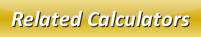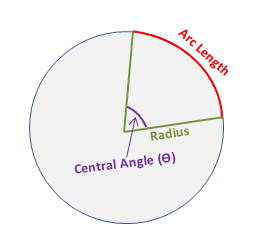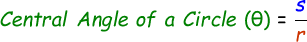# Central Angle of a Circle Calculator

Central angle is the angle that is formed by circle at the center by the 2 given points. Below is the an image which displays central angle of a circle:We can calculate the central angle of a circle with the help of this below formula:where,
Θ = Central Angle [radians]
s = Arc Length

Calculate central angle of a circle with the help of this below online calculator by entering the arc length and radius in the respective input boxes and click calculate button to find the central angle for the given circle.

 Arc Length (s): Radius (r): Central Angle (Θ): [radians]

Latest Calculator Release

Average Acceleration Calculator

Average acceleration is the object's change in speed for a specific given time period. ...

Free Fall Calculator

When an object falls into the ground due to planet's own gravitational force is known a...

Torque Calculator

Torque is nothing but a rotational force. In other words, the amount of force applied t...

Average Force Calculator

Average force can be explained as the amount of force exerted by the body moving at giv...

Angular Displacement Calculator

Angular displacement is the angle at which an object moves on a circular path. It is de...